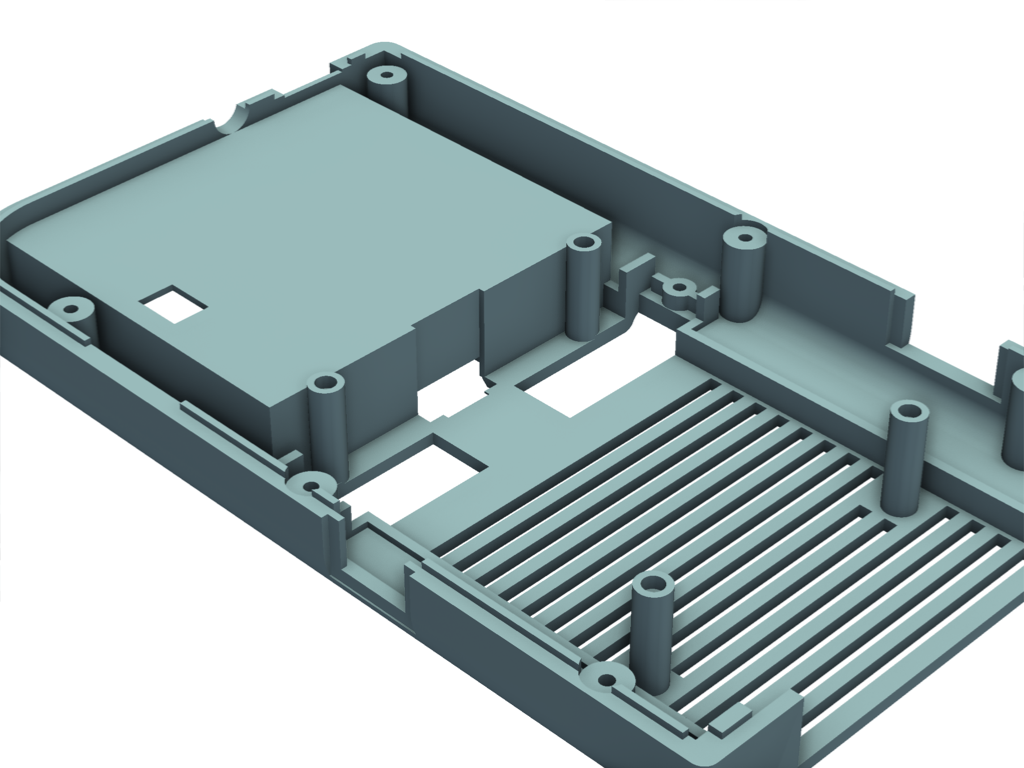# Surface Space Ambient Occlusion#

Demonstrate the usage of surface space ambient occlusion.

Surface space ambient occlusion (SSAO) can approximate shadows more efficiently than ray-tracing and produce similar results. Use this when you wish to plot the occlusion effect that nearby meshes have on each other by blocking nearby light sources.

See Kitware: Screen-Space Ambient Occlusion for more details

```# First, let's create several cubes nearby each other

import pyvista as pv
from pyvista import examples

grid = pv.UniformGrid(dimensions=(5, 5, 5)).explode(0.2)
```

# Plot with defaults#

Let’s plot this without SSAO. Note how the lighting is identical for each cube.

```pl = pv.Plotter()
pl.show()
```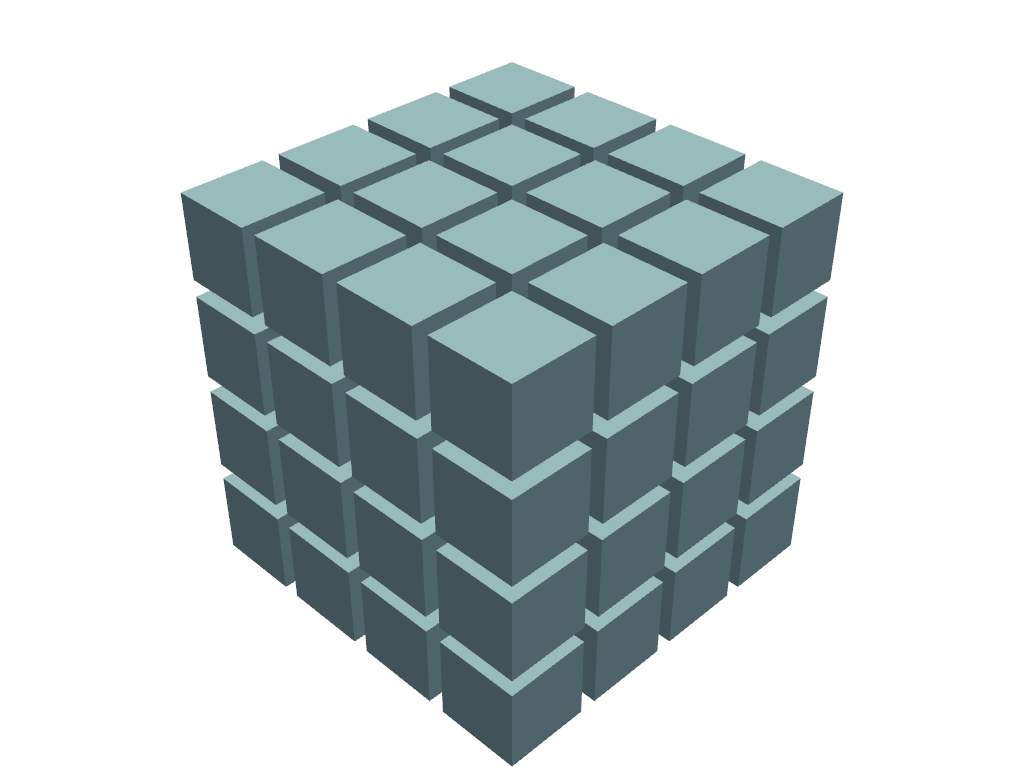# Plot with SSAO#

Now plot this with SSAO. Note how adjacent cubes affect the lighting of each other to make it look less artificial.

With a low `kernel_size`, the image will be rendered quickly at the expense of quality.

```pl = pv.Plotter()
pl.enable_ssao(kernel_size=32)
pl.show()
```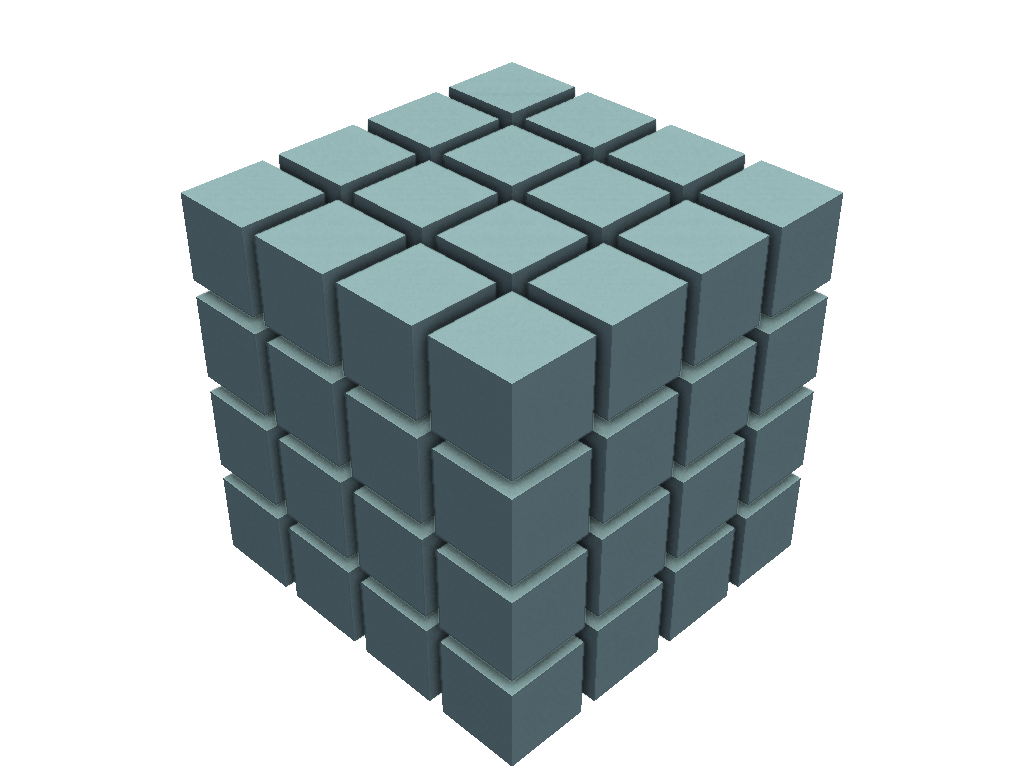# Improve the SSAO rendering#

Here we’ve increased the `kernel_size` to improve the quality of the SSAO and also enabled SSAA anti-aliasing to smooth out any of the artifacts created from SSAO.

```pl = pv.Plotter()
pl.enable_ssao(kernel_size=128)
pl.enable_anti_aliasing('ssaa')
pl.show()
```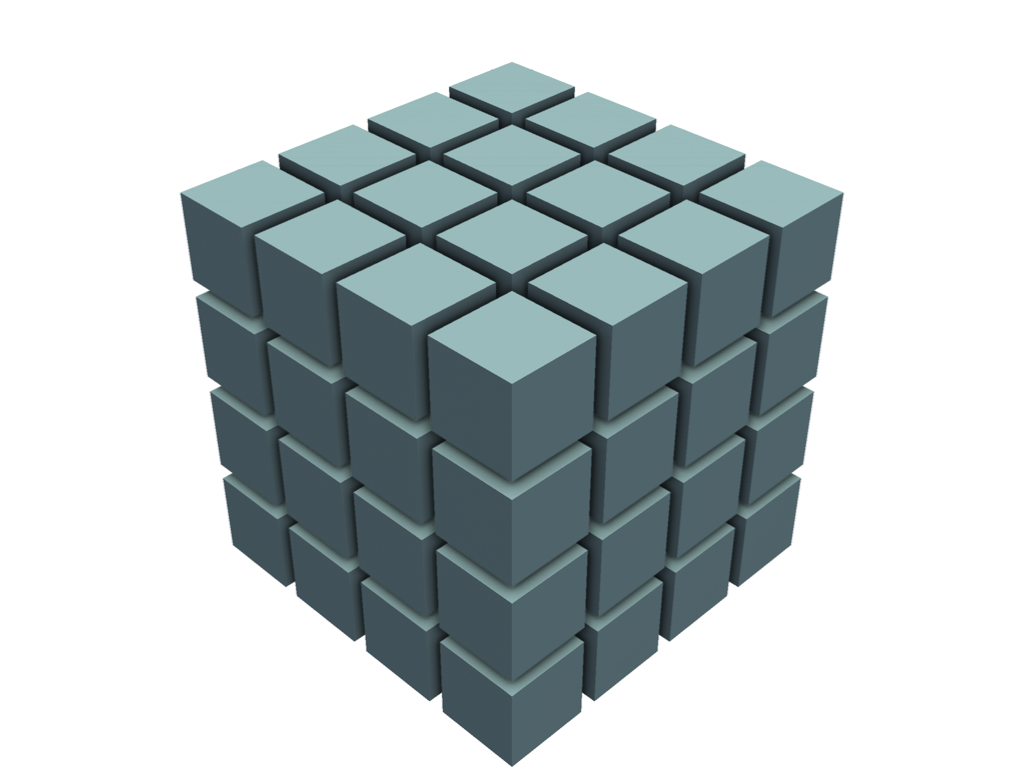# Plot a CAD model without SSAO#

Here’s another example without SSAO. This is a CAD model of a Raspberry PI case.

```mesh = examples.download_cad_model_case()

pl = pv.Plotter()
pl.enable_anti_aliasing('ssaa')
pl.camera.zoom(1.7)
pl.show()
```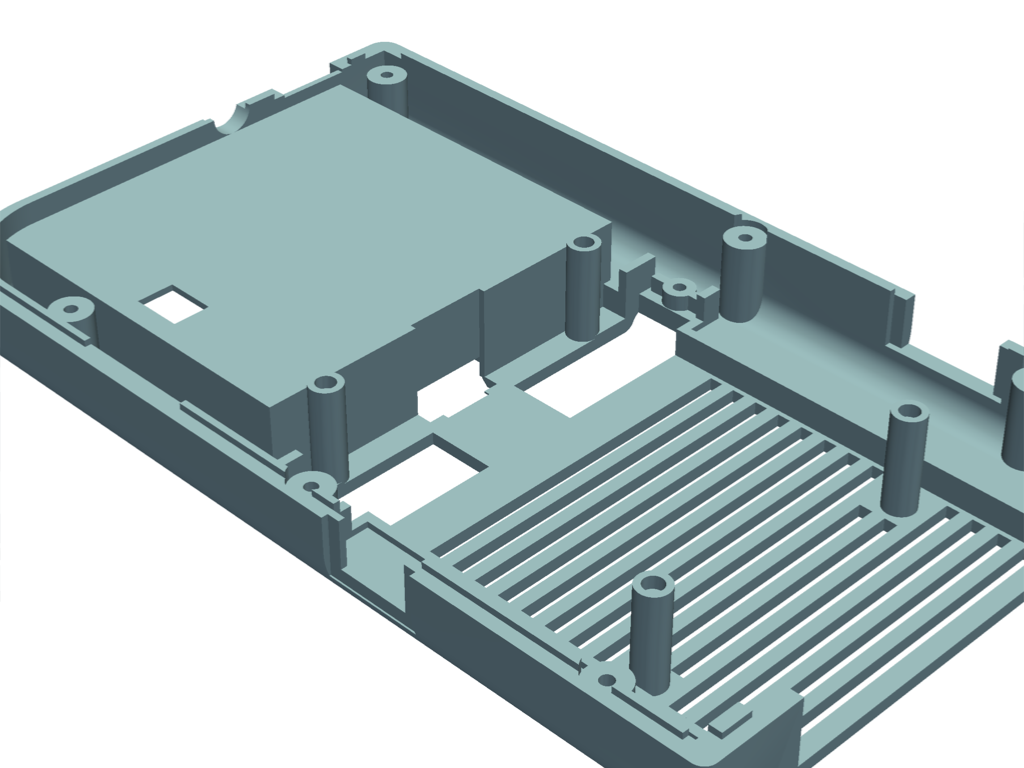# Plot with SSAO#

Here’s the same CAD model with SSAO. Note how we had to increase both `radius` and `bias` due to the relative scene size.

Note that the occlusion still seems quite small. In the next example we will increase the `radius` to increase the effect of the occlusion.

```pl = pv.Plotter()
pl.enable_anti_aliasing('ssaa')
pl.camera.zoom(1.7)
pl.show()
```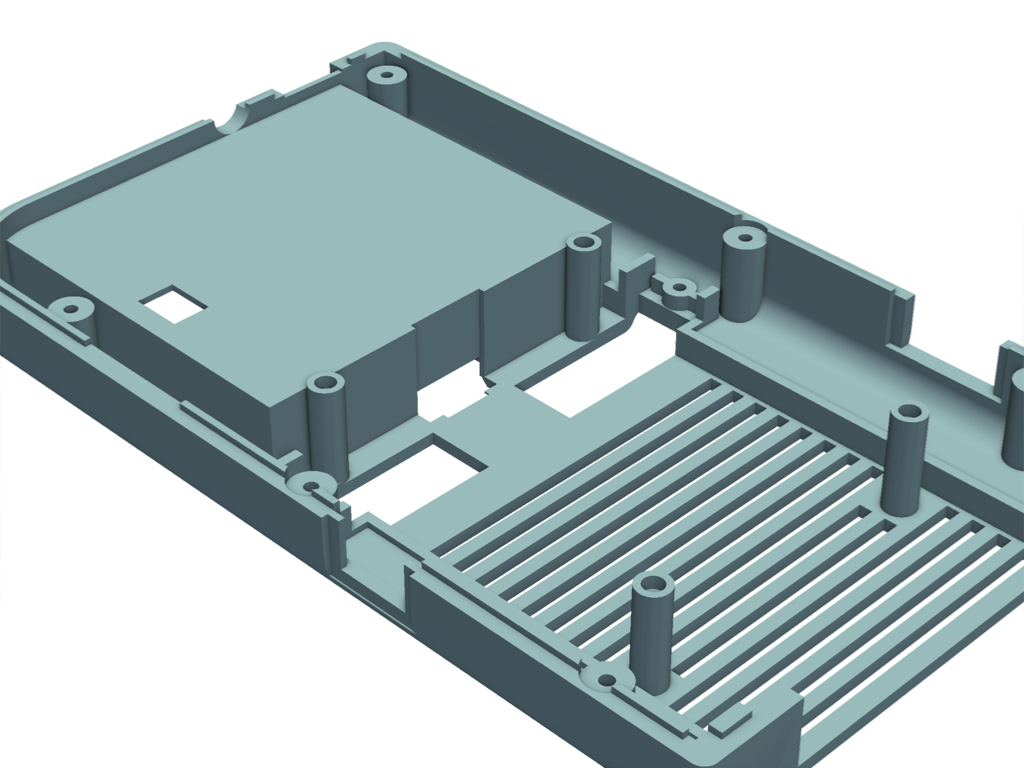Here we’ve increased the `radius` to the point where the case occlusion now seems realistic without it becoming overwhelming.

```pl = pv.Plotter()